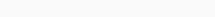# Math Puzzles Puzzle 45 Answer AndroidMath puzzle game level 45 solution with detailed hints and explanation, Scroll below to find out.

Math puzzles increase your logical thinking. The objective of the game is to find patterns hidden in the puzzle in order to arrive at the solution. Challenge yourself with different levels of math puzzles and stretch the limits of your intelligence.

### Math Puzzles Puzzle 45:

if 2,6=5

4,9=7

6,11=8

8,15=11

then 12,18=?Solution/Hint: (B-A/2)

18-12/2=12

If there is any Doubt or you are unable to understand the solution, then please let me know in comments, I will surely help you.

### One thought on “Math Puzzles Game Level 45 Answer with Solution”

•March 15, 2020 at 20:13